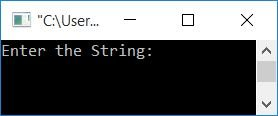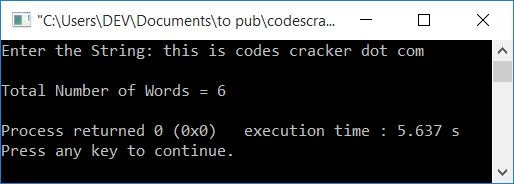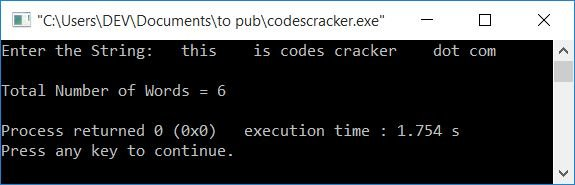# C++ Program to Count the Number of Words in a String

In this article, you will learn and get code to count the total number of words present or available in a given string by the user at run-time in the C++ language.

For example, if you enter "codes cracker dot com," the total number of words is four.

## In C++, count the total number of words in the string

To count the total number of words available in a string in C++ programming, you have to ask the user to enter the string or sentence first. And then count and print the result as shown in the program given below.

The question is, "Write a program in C++ that counts the total number of words in a string." Here is its answer.

```#include<iostream>
#include<stdio.h>
using namespace std;
int main()
{
char str;
int i=0, chk=0, countWord=0;
cout<<"Enter the String: ";
gets(str);
while(str[i]!='\0')
{
if(str[i]==' ')
{
if(chk!=0)
countWord++;
chk=0;
}
else
{
chk++;
}
i++;
}
if(chk!=0)
countWord++;
cout<<"\nTotal Number of Words = "<<countWord;
cout<<endl;
return 0;
}```

This program was built and runs under the Code::Blocks IDE. Here is its sample run:Now supply any string, say, this is codes cracker dot com as input, and press the ENTER key to count and print the total number of words available in the entered string:And here is another sample run with the following user input:

`  this    is codes cracker    dot com  `

That is, __this____is_codes_cracker____dot_com__. In this string, there are 2, 4, 1, 1, 4, and 1 spaces available before each word, and 2 spaces after the last word:The dry run of the above program with user input, this is codes cracker dot com, goes like this:

• Initial values: i=0, chk=0, countWord=0.
• When the user enters a string, then it gets stored in the str variable in a way that
• str=t
• str=h
• str=i
• and so on, up to
• str=m
• Because the maximum size of str[] is 200, but the entered string is of size 29 (because indexing starts at 0, so 28 (the last index) + 1 will be the size). Therefore, at the next index after the last character's index, a null-terminated character (\0) automatically gets assigned. So str=\0
• A null-terminated character indicates that no character is left in the index, where it is available.
• Now the condition of the while loop gets evaluated.
• That is, the condition str[i]!='\0' or str!='\0' or t!='\0' evaluates to be true, therefore program flow goes inside the loop.
• There, the condition of the if gets evaluated.
• That is, the condition str[i]==' ' or str==' ' or t==' ' evaluates to be false, therefore, program flow does not go inside its body, rather it goes to else's body and increments the value of chk. So chk=1
• And as in the last statement, the value of i gets incremented. So i=1
• Now program flow goes back and evaluates the condition of the while loop again.
• The process continues until the condition of the while loop evaluates to be false.
• On continuing the evaluation of the while loop, here are the values after each evaluation:
• chk=1, i=1
• chk=2, i=2
• chk=3, i=3
• chk=4, i=4
• chk=0, countWord=1, i=5
• chk=1, i=6
• chk=2, i=7
• chk=0, countWord=2, i=8
• chk=1, i=9
• chk=2, i=10
• chk=3, i=11
• chk=4, i=12
• chk=5, i=13
• chk=0, countWord=3, i=14
• chk=1, i=15
• chk=2, i=16
• chk=3, i=17
• chk=4, i=18
• chk=5, i=19
• chk=6, i=20
• chk=7, i=21
• chk=0, countWord=4, i=22
• chk=1, i=23
• chk=2, i=24
• chk=3, i=25
• chk=0, countWord=5, i=26
• chk=1, i=27
• chk=2, i=28
• chk=3, i=29
• After exiting from the while loop, the condition chk!=0 or 3!=0 evaluates to be true, so the value of countWord gets incremented. So countWord=6
• Now print the value of countWord as the output of the total number of words in the string.

C++ Quiz

« Previous Program Next Program »